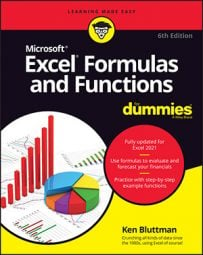##### Excel Formulas & Functions For DummiesIt’s easy to use Excel for many of your day-to-day number-crunching tasks, like determining your business’s average sale, computing classroom grades, or forecasting college expenses. Use this handy Cheat Sheet to discover great functions and tips to help you get the most out of Excel.

## Excel functions you're likely to use

Some Excel functions apply to specific subject areas, but others are general and apply to all needs. The following list shows an array of Excel functions used by one and all. Check here for a quickie reference to the purpose of each Excel function.

Excel Function Description
SUM Calculates the sum of a group of values
AVERAGE Calculates the mean of a group of values
COUNT Counts the number of cells in a range that contains
numbers
INT Removes the decimal portion of a number, leaving just the
integer portion
ROUND Rounds a number to a specified number of decimal places or
digit positions
IF Tests for a true or false condition and then returns one value
or another
NOW Returns the system date and time
TODAY Returns the system date, without the time
SUMIF Calculates a sum from a group of values, but just of values
that are included because a condition is met
COUNTIF Counts the number of cells in a range that match a
criteria

## Excel text functions you'll find helpful

Excel’s text functions are very helpful when you’re working with names, addresses, customer lists, or any other text-based data. Here is list of Excel functions associated with text, along with a description of what each function does:

Function Description
LEFT Extracts one or more characters from the left side of a text
string
RIGHT Extracts one or more characters from the right side of a text
string
MID Extracts characters from the middle of a text string; you
specify which character position to start from and how many
characters to include
CONCATENATE Assembles two or more text strings into one
REPLACE Replaces part of a text string with other text
LOWER Converts a text string to all lowercase
UPPER Converts a text string to all uppercase
PROPER Converts a text string to proper case
LEN Returns a text string’s length (number of
characters)

## Excel order of operations to keep in mind

Mathematics dictates a protocol of how formulas are interpreted, and Excel follows that protocol. The following is the order in which mathematical operators and syntax are applied both in Excel and in general mathematics. You can remember this order by memorizing the mnemonic phrase, “Please excuse my dear aunt Sally.”

1. Parentheses

2. Exponents

3. Multiplication and division

## Excel references worth remembering

In Excel formulas, you can refer to other cells either relatively or absolutely. When you copy and paste a formula in Excel, how you create the references within the formula tells Excel what to change in the formula it pastes. The formula can either change the references relative to the cell where you’re pasting it (relative reference), or it can always refer to a specific cell.

You can also mix relative and absolute references so that, when you move or copy a formula, the row changes but the column does not, or vice versa.

Preceding the row and/or column designators with a dollar sign (\$) specifies an absolute reference in Excel.

Example Comment
=A1 Complete relative reference
=\$A1 The column is absolute; the row is relative
=A\$1 The column is relative; the row is absolute
=\$A\$1 Complete absolute reference

## Excel error messages to get to know

If you create a formula in Excel that contains an error or circular reference, Excel lets you know about it with an error message. A handful of errors can appear in a cell when a formula or function in Excel cannot be resolved. Knowing their meaning helps correct the problem.

Error Meaning
#DIV/0! Trying to divide by 0
#N/A! A formula or a function inside a formula cannot find the
referenced data
#NAME? Text in the formula is not recognized
#NULL! A space was used in formulas that reference multiple ranges; a
comma separates range references
#NUM! A formula has invalid numeric data for the type of
operation
#REF! A reference is invalid
#VALUE! The wrong type of operand or function argument is used